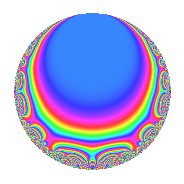Properties

 Label 24.3.bLevel 24 Weight 3 Character orbit b Rep. character $$\chi_{24}(19,\cdot)$$ Character field $$\Q$$ Dimension 4 Newforms 1 Sturm bound 12 Trace bound 0

Related objects

Defining parameters

 Level: $$N$$ = $$24 = 2^{3} \cdot 3$$ Weight: $$k$$ = $$3$$ Character orbit: $$[\chi]$$ = 24.b (of order $$2$$ and degree $$1$$) Character conductor: $$\operatorname{cond}(\chi)$$ = $$8$$ Character field: $$\Q$$ Newforms: $$1$$ Sturm bound: $$12$$ Trace bound: $$0$$

Dimensions

The following table gives the dimensions of various subspaces of $$M_{3}(24, [\chi])$$.

Total New Old
Modular forms 10 4 6
Cusp forms 6 4 2
Eisenstein series 4 0 4

Trace form

 $$4q$$ $$\mathstrut +\mathstrut 2q^{2}$$ $$\mathstrut -\mathstrut 8q^{4}$$ $$\mathstrut -\mathstrut 6q^{6}$$ $$\mathstrut -\mathstrut 4q^{8}$$ $$\mathstrut +\mathstrut 12q^{9}$$ $$\mathstrut +\mathstrut O(q^{10})$$ $$4q$$ $$\mathstrut +\mathstrut 2q^{2}$$ $$\mathstrut -\mathstrut 8q^{4}$$ $$\mathstrut -\mathstrut 6q^{6}$$ $$\mathstrut -\mathstrut 4q^{8}$$ $$\mathstrut +\mathstrut 12q^{9}$$ $$\mathstrut +\mathstrut 12q^{10}$$ $$\mathstrut -\mathstrut 32q^{11}$$ $$\mathstrut -\mathstrut 12q^{12}$$ $$\mathstrut +\mathstrut 36q^{14}$$ $$\mathstrut -\mathstrut 8q^{16}$$ $$\mathstrut -\mathstrut 8q^{17}$$ $$\mathstrut +\mathstrut 6q^{18}$$ $$\mathstrut +\mathstrut 32q^{19}$$ $$\mathstrut +\mathstrut 72q^{20}$$ $$\mathstrut -\mathstrut 16q^{22}$$ $$\mathstrut +\mathstrut 36q^{24}$$ $$\mathstrut -\mathstrut 44q^{25}$$ $$\mathstrut -\mathstrut 96q^{26}$$ $$\mathstrut -\mathstrut 48q^{28}$$ $$\mathstrut -\mathstrut 60q^{30}$$ $$\mathstrut -\mathstrut 88q^{32}$$ $$\mathstrut +\mathstrut 44q^{34}$$ $$\mathstrut +\mathstrut 96q^{35}$$ $$\mathstrut -\mathstrut 24q^{36}$$ $$\mathstrut +\mathstrut 40q^{38}$$ $$\mathstrut +\mathstrut 120q^{40}$$ $$\mathstrut +\mathstrut 40q^{41}$$ $$\mathstrut +\mathstrut 84q^{42}$$ $$\mathstrut +\mathstrut 32q^{43}$$ $$\mathstrut +\mathstrut 64q^{44}$$ $$\mathstrut -\mathstrut 72q^{46}$$ $$\mathstrut +\mathstrut 96q^{48}$$ $$\mathstrut -\mathstrut 44q^{49}$$ $$\mathstrut -\mathstrut 118q^{50}$$ $$\mathstrut -\mathstrut 96q^{51}$$ $$\mathstrut -\mathstrut 48q^{52}$$ $$\mathstrut -\mathstrut 18q^{54}$$ $$\mathstrut -\mathstrut 168q^{56}$$ $$\mathstrut -\mathstrut 48q^{57}$$ $$\mathstrut +\mathstrut 156q^{58}$$ $$\mathstrut -\mathstrut 128q^{59}$$ $$\mathstrut -\mathstrut 96q^{60}$$ $$\mathstrut +\mathstrut 204q^{62}$$ $$\mathstrut -\mathstrut 32q^{64}$$ $$\mathstrut +\mathstrut 96q^{65}$$ $$\mathstrut +\mathstrut 48q^{66}$$ $$\mathstrut -\mathstrut 256q^{67}$$ $$\mathstrut +\mathstrut 112q^{68}$$ $$\mathstrut +\mathstrut 24q^{70}$$ $$\mathstrut -\mathstrut 12q^{72}$$ $$\mathstrut +\mathstrut 200q^{73}$$ $$\mathstrut -\mathstrut 120q^{74}$$ $$\mathstrut +\mathstrut 192q^{75}$$ $$\mathstrut -\mathstrut 16q^{76}$$ $$\mathstrut -\mathstrut 48q^{78}$$ $$\mathstrut -\mathstrut 96q^{80}$$ $$\mathstrut +\mathstrut 36q^{81}$$ $$\mathstrut -\mathstrut 124q^{82}$$ $$\mathstrut +\mathstrut 160q^{83}$$ $$\mathstrut -\mathstrut 24q^{84}$$ $$\mathstrut +\mathstrut 88q^{86}$$ $$\mathstrut +\mathstrut 32q^{88}$$ $$\mathstrut -\mathstrut 200q^{89}$$ $$\mathstrut +\mathstrut 36q^{90}$$ $$\mathstrut +\mathstrut 288q^{91}$$ $$\mathstrut +\mathstrut 96q^{92}$$ $$\mathstrut -\mathstrut 168q^{94}$$ $$\mathstrut -\mathstrut 24q^{96}$$ $$\mathstrut +\mathstrut 56q^{97}$$ $$\mathstrut +\mathstrut 170q^{98}$$ $$\mathstrut -\mathstrut 96q^{99}$$ $$\mathstrut +\mathstrut O(q^{100})$$

Decomposition of $$S_{3}^{\mathrm{new}}(24, [\chi])$$ into irreducible Hecke orbits

Label Dim. $$A$$ Field CM Traces $q$-expansion
$$a_2$$ $$a_3$$ $$a_5$$ $$a_7$$
24.3.b.a $$4$$ $$0.654$$ 4.0.4752.1 None $$2$$ $$0$$ $$0$$ $$0$$ $$q+(\beta _{1}-\beta _{2})q^{2}+\beta _{2}q^{3}+(-2-\beta _{2}+\cdots)q^{4}+\cdots$$

Decomposition of $$S_{3}^{\mathrm{old}}(24, [\chi])$$ into lower level spaces

$$S_{3}^{\mathrm{old}}(24, [\chi]) \cong$$ $$S_{3}^{\mathrm{new}}(8, [\chi])$$$$^{\oplus 2}$$